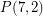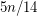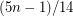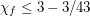# Does every subcubic triangle-free graph have fractional chromatic number at most 14/5? (Solved)

 Importance: Low ✭
 Author(s): Heckman, Christopher C. Thomas, Robin
 Subject: Graph Theory » Coloring » » Vertex coloring
 Keywords: fractional coloring
 Posted by: Andrew King on: April 20th, 2011
 Solved by: Zdeněk Dvořák, Jean-Sébastien Sereni, and Jan Volec [DSV]
Conjecture   Every triangle-free graph with maximum degree at most 3 has fractional chromatic number at most 14/5.

This conjecture was posed by Heckman and Thomas [HT]. Due to the generalized Petersen graph, which has 14 vertices and no stable set of size 6, this would be best possible.

The conjecture requires that every triangle-free subcubic graph contains a stable set of size at least. This was first proved by Staton [S]. The proof was then improved Jones [J]. Griggs and Murphy [GM] gave a linear-time algorithm for finding a stable set of size at leastfor any connected subcubic graph, and Heckman and Thomas [HT] have a linear-time algorithm to find a stable set of size at least.

Hatami and Zhu [HZ] proved that triangle-free subcubic graphs have fractional chromatic number at most. Lu and Peng [LP] improved this bound to.

## Bibliography

**[DSV] Z. Dvořák, J.-S. Sereni, and J. Volec, Subcubic triangle-free graphs have fractional chromatic number at most 14/5, arXiv:1301.5296 [math.CO]

[GM] J. Griggs and O. Murphy. Edge density and the independence ratio in triangle-free graphs with maximum degree three. Discrete Math. 152 (1996) 157--170.

[HZ] H. Hatami and X. Zhu. The fractional chromatic number of graphs of maximum degree at most three. SIAM J. Discrete Math. 233 (2001) 233-237.

*[HT] Christopher Carl Heckman and Robin Thomas. A new proof of the independence ratio of triangle-free cubic graphs. Discrete Math. 233 (2001), 233--237.

[J] K. F. Jones. Size and independence in triangle-free graphs with maximum degree three. J. Graph Theory 14 (1990) 525--535.

[S] W. Staton. SOme Ramsey-type numbers and the independence ratio. Trans. Amer. Math. Soc. 256 (1979) 353--370.

* indicates original appearance(s) of problem.

### This conjecture has recently

This conjecture has recently been proved by Dvorak, Sereni and Volec.

Frederic Havet